# NCERT Solutions for Class 7 Maths Chapter 10 Practical Geometry (EX 10.2) Exercise 10.2

## NCERT Solutions for Class 7 Maths Chapter 10 Practical Geometry (EX 10.2) Exercise 10.2

Free PDF download of NCERT Solutions for Class 7 Maths Chapter 10 Exercise 10.2 (EX 10.2) and all chapter exercises at one place prepared by expert teacher as per NCERT (CBSE) books guidelines. Class 7 Maths Chapter 10 Practical Geometry Exercise 10.2 Questions with Solutions to help you to revise complete Syllabus and Score More marks. Register and get all exercise solutions in your emails.

Do you need help with your Homework? Are you preparing for Exams?
Study without Internet (Offline)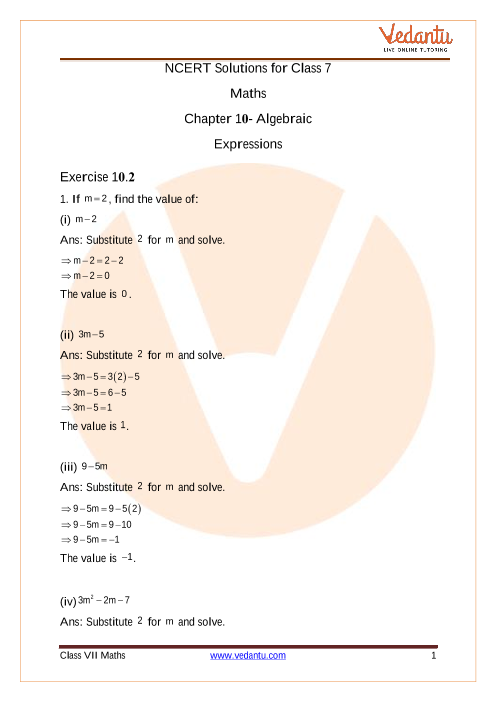Book your Free Demo session
Get a flavour of LIVE classes here at Vedantu## Access NCERT Solutions for Class 7 Maths Chapter 10- Practical Geometry

Exercise 10.2

Refer to pages 6-7 for exercise 10.2 in the PDF

1. Construct $\vartriangle XYZ$ in which $XY = 4.5$cm, $YZ = 5$cm and $ZX = 6$cm.

Ans: To construct: $\vartriangle XYZ$, where $XY = 4.5$cm, $YZ = 5$cm and $ZX = 6$cm.

Steps of construction:

(a) Draw a line segment $YZ = 5$cm.

(b) Take $Z$ as centre and radius as $6$cm, draw an arc.

(c) Similarly, taking $Y$ as centre and radius $4.5$cm, mark another arc that intersects the first arc at point $X$.

(d) Join $XY$ and $XZ$.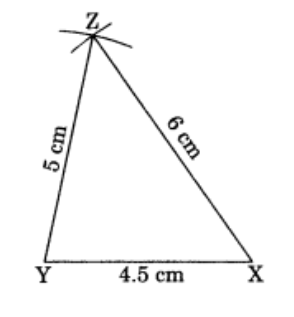This is the required $\vartriangle XYZ$.

2. Construct an equilateral triangle of 5.5 cm.

Ans: To construct: A $\vartriangle ABC$ where $AB = BC = CA = 5.5$cm.

Steps of construction:

(a) Draw a line segment $BC = 5.5$cm.

(b) Taking points $B$ and $C$ as centres and radius $5.5$cm, draw arcs which intersect at point $A$.

(c) Join $AB$ and $AC$.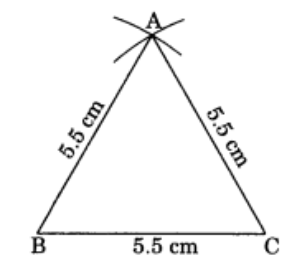This is the required $\vartriangle ABC$.

3. Draw $\vartriangle PQR$ with $PQ = 4$cm, $QR = 3.5$cm and $PR = 4$cm. What type of triangle is this?

Ans: To construction: $\vartriangle PQR$, in which $PQ = 4$cm, $QR = 3.5$cm and $PR = 4$cm.

Steps of construction:

(a) Draw a line segment $QR = 3.5$cm.

(b) Take $Q$ as centre and radius as $4$cm and draw an arc.

(c) Similarly, take $R$ as centre and radius as $4$cm and draw another arc which intersects the first arc at $P$.

(d) Join $PQ$ and $PR$.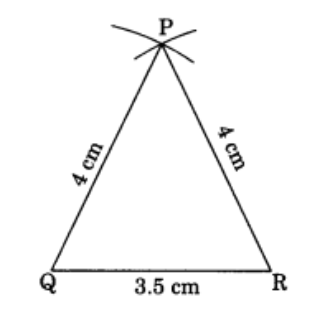The triangle obtained is the isosceles $\vartriangle PQR$.

4. Construct $\vartriangle ABC$ such that $AB = 2.5$cm, $BC = 6$cm and $AC = 6.5$cm. Measure $\angle B$.

Ans: To construct: $\vartriangle ABC$ in which $AB = 2.5$cm, $BC = 6$cm and $AC = 6.5$cm.

Steps of construction:

(a) Draw a line segment $BC = 6$cm.

(b) Taking $B$ as centre and radius $2.5$cm, draw an arc.

(c) Similarly, take $C$ as centre and radius $6.5$cm then draw another arc which intersects the first arc at $A$.

(d) Join $AB$ and $AC$.

(e) Now measure angle $B$ with the help of a protractor.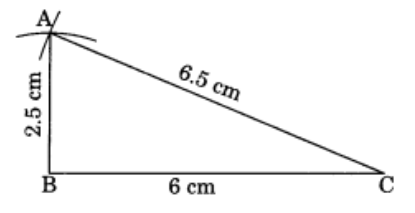It is the required $\vartriangle ABC$ where $\angle B = {90^ \circ }$.

## NCERT Solutions for Class 7 Maths Chapter 10 Practical Geometry Exercise 10.2

Opting for the NCERT solutions for Ex 10.2 Class 7 Maths is considered as the best option for the CBSE students when it comes to exam preparation. This chapter consists of many exercises. Out of which we have provided the Exercise 10.2 Class 7 Maths NCERT solutions on this page in PDF format. You can download this solution as per your convenience or you can study it directly from our website/ app online.

Vedantu in-house subject matter experts have solved the problems/ questions from the exercise with the utmost care and by following all the guidelines by CBSE. Class 7 students who are thorough with all the concepts from the Maths textbook and quite well-versed with all the problems from the exercises given in it, then any student can easily score the highest possible marks in the final exam. With the help of this Class 7 Maths Chapter 10 Exercise 10.2 solutions, students can easily understand the pattern of questions that can be asked in the exam from this chapter and also learn the marks weightage of the chapter. So that they can prepare themselves accordingly for the final exam.

Besides these NCERT solutions for Class 7 Maths Chapter 10 Exercise 10.2, there are plenty of exercises in this chapter which contain innumerable questions as well. All these questions are solved/answered by our in-house subject experts as mentioned earlier. Hence all of these are bound to be of superior quality and anyone can refer to these during the time of exam preparation. In order to score the best possible marks in the class, it is really important to understand all the concepts of the textbooks and solve the problems from the exercises given next to it.

Do not delay any more. Download the NCERT solutions for Class 7 Maths Chapter 10 Exercise 10.2 from Vedantu website now for better exam preparation. If you have the Vedantu app in your phone, you can download the same through the app as well. The best part of these solutions is these can be accessed both online and offline as well.SHARETWEETSHARESUBSCRIBE